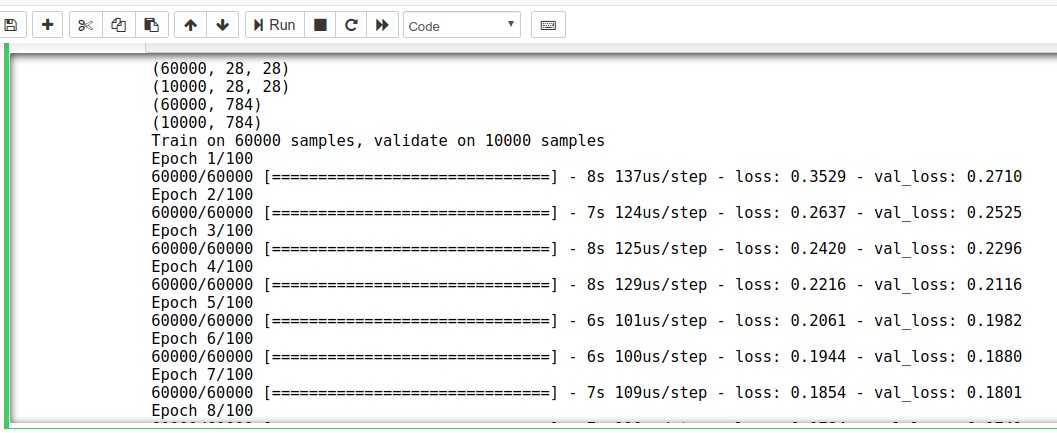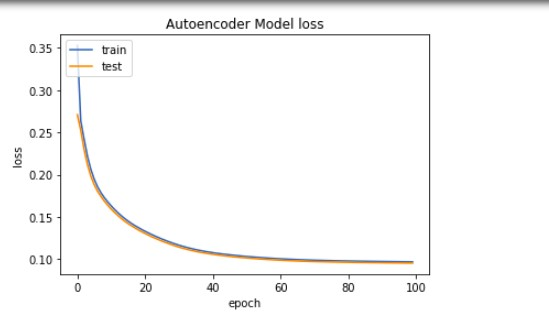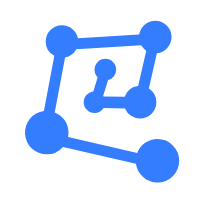# Community

Blog Events Webinars Tutorials Forum
×
Community Blog Implementing Reinforcement Learning with Keras

# Implementing Reinforcement Learning with Keras

In this tutorial, you will be learning how you can implement your own reinforcement learning tasks on Alibaba Cloud's Machine Learning Platform with Keras.

By Oluwabukunmi Ige, Alibaba Cloud Community Blog author.

Alibaba Cloud's machine learning platform provides its customers with some powerful GPUs that are fully capable of performing some impressive deep learning and reinforcement learning tasks. In this tutorial, I will discuss how you can implement your own reinforcement learning tasks on Alibaba Cloud's Machine Learning Platform.

Before we get into the main part of this tutorial, let's first cover some important concepts:

Reinforcement Learning (RL) is a type of machine learning algorithm that trains algorithms based on a mechanism in which certain actions are associated with certain rewards.

RL approbates the concept of infants interacting with their environment, performing actions, drawing intuitions and learning from experience with limited human input. The model employs a trial-and-error method that is based on a reward-and-penalty system. That is, the model learns by trying all possible routes and then selecting the route that gives a reward with the least possible penalties.

RL comes into play when there is no hard-coded method for performing a task, but rather there are some set of rules that need to be followed in order for a model to achieve its desired objectives. RL as a machine learning algorithm models how humans learn and has been predicted as being pivotal in attaining Artificial General Intelligence in AI-based applications.

Keras is an open-source neural network library written in Python. Keras runs on a high-level API that handles the way models are built, layers are defined or set up in multiple inputs and output models. Keras outsources its low-level API tasks like making tensors and computational graphs, so on, to its backend engine. Keras is generally preferred in reinforcement learning scenarios because it is easy to understand, fast to deploy, has a large community that supports it, has support for multiple backends, and it is easy to implement on many different platforms, including iOS, Android, and desktop browsers.

In this tutorial, we will specifically be using Reinforcement learning concepts to build a digit image recognizer. The dataset that we will be using is MNIST dataset available in the `keras.datasets` module. The model will be trained on an Alibaba Cloud GPU running on a Jupyter Notebook.

## Requirements for This Tutorial

The prerequisites to building this RL model on Alibaba Cloud instance are as follows:

1. An Alibaba Cloud Elastic Compute Service (ECS) instance with Ubuntu 16.04 and 10GB RAM on an NVIDIA GPU.
2. A root password setup on the server
3. Anaconda running on your Alibaba Cloud instance. Anaconda is an open-source package manager that contains a number of Python modules and packages, including, the one important for this tutorial, Jupyter Notebook, which lets you build, visualize and train your machine learning and deep learning models.
4. The Keras library installed on your Jupyter notebook.

To get ready for the rest of this tutorial,can complete the prerequisites given above, you'll want to first complete these steps:

• Log in to the Alibaba Cloud ECS console and create a new ECS instance. Choose Ubuntu 16.04 as your operating system and at least 10GB RAM GPU NVIDIA. Make sure you choose China (Shanghai) region as your location as that's the only location that supports GPU clusters for now on Alibaba Cloud. Check the Auto-install GPU driver icon to automatically install CUDA and GPU driver when the instance is being created.
• Initiate Anaconda on your Alibaba Cloud ECS Console. If you don't have it installed you can follow this tutorial to install it and launch it.
• Launch your Jupyter Notebook instance.
• Keras usually comes pre-installed in Jupyter notebook, but if it is not installed on your instance, you'll need to install it. You can install it using `! pip install keras` or `conda install -c conda-forge keras` (recommended) from your command line interface terminal.

## Build and Train the Reinforcement Learning Model

After you've completed all of the steps above, the next step for you to do is to build and train the model. The code snippets below will show a step-by-step analysis of how the model is being built and trained. You'll want to run each code block by pressing shift + enter in your Jupyter Notebook.

• First, import the needed libraries.
``````from keras.layers import Input, Dense
from keras.models import Model
import numpy as np
import matplotlib.pyplot as plt``````
• Next, load the data and split into train and test sets.
``````from keras.datasets import mnist
(x_train, y_train), (x_test, y_test) =``````
• Last, normalize and reshape the data before ingestion into the model.
``````x_train = x_train.astype('float32') / 255
x_test = x_test.astype('float32') / 255
x_train = x_train.reshape((len(x_train), np.prod(x_train.shape[1:])))
x_test = x_test.reshape((len(x_test), np.prod(x_test.shape[1:])))``````

## Setting up the Model Architecture

The next few steps are related to figuring out your model architecture:

• First, you'll want to define the input.
``InputModel = Input(shape=(784,))``
• Next, you'll want to add the encoding layer where your activation fuction is `RELU`.
``````EncodedLayer = Dense(32,
activation='relu')(InputModel)``````
• Next, you'll want to add the decoding layer and specify your activation function as `SIGMOID`.
``DecodedLayer = Dense(784, activation='sigmoid')(EncodedLayer)``
• Now, for this step, you'll want to define your auto-encoder model, compile it and fit to your train dataset. The fit method trains the model for a fixed number of epochs. After all of this is done, your model is ready.
``````AutoencoderModel = Model(InputModel, DecodedLayer)

history = AutoencoderModel.fit(x_train, x_train,
batch_size=256,
epochs=100,
shuffle=True,
validation_data=(x_test, x_test))``````
• Now, later on, you will use the model to rebuild the handwritten digits. To do this, you will use the predict method. This method generates output predictions for the input sample (`x_test`).
``DecodedDigits = AutoencoderModel.predict(x_test)``
• By running this code block, you will see a message for each of the 100 epochs, printing the loss and accuracy for each before a final evaluation of the trained model on the validation set. The screenshot below shows the training loss and `val_loss` for each epoch. It trains until it gets the loss as close as possible to the train set.• To have an idea of how the loss function varies during the epochs we can create a plot of the loss on the training and validation datasets during each epoch. The code block to visualize this is given below.
``````plt.plot(history.history['loss'])
plt.plot(history.history['val_loss'])
plt.title('Autoencoder Model loss')
plt.ylabel('loss')
plt.xlabel('epoch')
plt.legend(['train', 'test'], loc='upper left')
plt.show()``````• Finally, you want to verify your results:
``````n=10
plt.figure(figsize=(20, 4))
for i in range(n):
ax = plt.subplot(2, n, i + 1)
plt.imshow(x_test[i].reshape(28, 28))
plt.gray()
ax.get_xaxis().set_visible(False)
ax.get_yaxis().set_visible(False)
ax = plt.subplot(2, n, i + 1 + n)
plt.imshow(DecodedDigits[i].reshape(28, 28))
plt.gray()
ax.get_xaxis().set_visible(False)
ax.get_yaxis().set_visible(False)
plt.show()``````From the image above, you can see that your model has been able to generate entirely new handwriting images close to the original using the Auto-encoders Reinforcement Learning technique.

## Conclusion

In this tutorial, you've learn a bit about the concept of reinforcement learning and how you can implement it on Alibaba Cloud. Next, through this tutorial, you were able to have a reinforcement learning model built to generate a handwritten image. In many ways, this is just one simple example of how you can leverage Alibaba Cloud's powerful architecture for any of your Machine Learning tasks. In reality, the limits of this technology are only your imagination.

1 0 0
Share on

### You may also like## walid September 14, 2020 at 1:03 am

Hello,Please can you explain where are the (state, action, reward, environment, policy) in this technique, because I just understood that you used the autoencoder approach to train the model.

# Related Products

• ##ECS(Elastic Compute Service)

An online computing service that offers elastic and secure virtual cloud servers to cater all your cloud hosting needs.

• ##Machine Learning Platform For AI

An end-to-end platform that provides various machine learning algorithms to meet your data mining and analysis requirements.